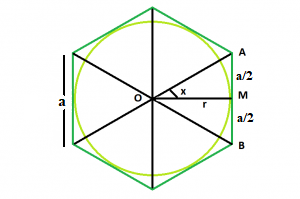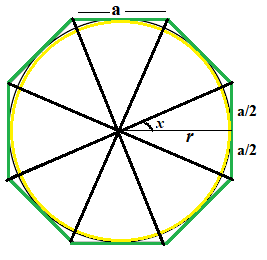# Area of largest Circle inscribe in N-sided Regular polygon

Given a regular polygon of N sides with side length a. The task is to find the area of the Circle which inscribed in the polygon.

Note : This problem is mixed version of This and This
Examples:

```Input: N = 6, a = 4
Output: 37.6801
Explanataion:In this, the polygon have 6 faces
and as we see in fig.1 we clearly see
that the angle  x  is 30 degree
so the radius of circle will be ( a / (2  * tan(30)))
Therefore, r = a√3/2

Input: N = 8, a = 8
Output: 292.81
Explanataion:In this, the polygon have 8 faces
and as we see in fig.2 we clearly see
that the angle  x  is 22.5 degree
so the radius of circle will be ( a / (2  * tan(22.5)))
Therefore, r = a/0.828
```

## Recommended: Please try your approach on {IDE} first, before moving on to the solution.

Approach: In the figure above, we see the polygon can be divided into N equal triangles. Looking into one of the triangles, we see that the whole angle at the center can be divided into = 360/N

So, angle x = 180/n
Now, tan(x) = (a / 2) * r

So, r = a / ( 2 * tan(x))

So, Area of the Inscribed Circle is,

``` A = Πr² = Π * (a / (2 * tan(x))) * (a / (2*tan(x)))
```

Below is the implementation of the above approach:

## C++

 `// C++ Program to find the area of a circle in ` `// inscribed in polygon ` ` `  `#include ` `using` `namespace` `std; ` ` `  `// Function to find the area ` `// of a circle ` `float` `InscribedCircleArea(``float` `n, ``float` `a) ` `{ ` `    ``// Side and side length cannot be negative ` `    ``if` `(a < 0 && n < 0) ` `        ``return` `-1; ` ` `  `    ``// degree converted to radians ` `    ``float` `r = a / (2 * ``tan``((180 / n) * 3.14159 / 180)); ` ` `  `    ``// area of circle ` `    ``float` `Area = (3.14) * (r) * (r); ` ` `  `    ``return` `Area; ` `} ` ` `  `// Driver code ` `int` `main() ` `{ ` ` `  `    ``// no.  of sides ` `    ``float` `n = 6; ` ` `  `    ``// side length ` `    ``float` `a = 4; ` ` `  `    ``cout << InscribedCircleArea(n, a) << endl; ` ` `  `    ``return` `0; ` `} `

## Java

 `// Java Program to find the area of a circle ` `// inscribed in a polygon ` `import` `java.io.*; ` ` `  `class` `GFG { ` ` `  `    ``// Function to find the area ` `    ``// of a regular polygon ` `    ``static` `float` `InscribedCircleArea(``float` `n, ``float` `a) ` `    ``{ ` `        ``// Side and side length cannot be negative ` `        ``if` `(a < ``0` `&& n < ``0``) ` `            ``return` `-``1``; ` ` `  `        ``// degree converted to radians ` `        ``float` `r = a / (``float``)(``2` `* Math.tan((``180` `/ n) * ``3.14159` `/ ``180``)); ` ` `  `        ``// area of circle ` `        ``float` `Area = (``float``)(``3.14``) * (r) * (r); ` ` `  `        ``return` `Area; ` `    ``} ` ` `  `    ``// Driver code ` ` `  `    ``public` `static` `void` `main(String[] args) ` `    ``{ ` ` `  `        ``// no.  of sides ` `        ``float` `n = ``6``; ` ` `  `        ``// side length ` `        ``float` `a = ``4``; ` ` `  `        ``System.out.println(InscribedCircleArea(n, a)); ` `    ``} ` `} `

## Python3

 `# Python 3 Program to find the area  ` `# of a circle inscribed ` `# in a polygon ` `from` `math ``import` `tan ` ` `  `# Function to find the area of a  ` `# circle ` `def` `InscribedCircleArea(n, a): ` `    ``# Side and side length cannot  ` `    ``# be negative ` `    ``if` `(a < ``0` `and` `n < ``0``): ` `        ``return` `-``1` ` `  `    ``# degree converted to radians ` `    ``r ``=` `a``/``(``2` `*` `tan((``180` `/` `n) ``*` `3.14159` `/` `180``)); ` ` `  `    ``# area of circle ` `    ``Area ``=` `3.14` `*` `r ``*` `r ` ` `  `    ``return` `Area ` ` `  `# Driver code ` `if` `__name__ ``=``=` `'__main__'``: ` `    ``a ``=` `4` `    ``n ``=` `6` ` `  `    ``print``(``'{0:.6}'``.``format``(InscribedCircleArea(n, a))) ` ` `  `# This code is contributed by ` `# Chandan Agrawal `

## C#

 `// C# Program to find the area of a circle  ` `// inscribed in a polygon  ` `using` `System; ` ` `  `class` `GFG  ` `{  ` ` `  `// Function to find the area  ` `// of a regular polygon  ` `static` `float` `InscribedCircleArea(``float` `n, ``float` `a)  ` `{  ` `    ``// Side and side length cannot be negative  ` `    ``if` `(a < 0 && n < 0)  ` `        ``return` `-1;  ` ` `  `    ``// degree converted to radians  ` `    ``float` `r = a / (``float``)(2 * Math.Tan((180 / n) *  ` `                                 ``3.14159 / 180));  ` ` `  `    ``// area of circle  ` `    ``float` `Area = (``float``)(3.14) * (r) * (r);  ` ` `  `    ``return` `Area;  ` `}  ` ` `  `// Driver code  ` `public` `static` `void` `Main()  ` `{  ` ` `  `    ``// no. of sides  ` `    ``float` `n = 6;  ` ` `  `    ``// side length  ` `    ``float` `a = 4;  ` ` `  `    ``Console.WriteLine(InscribedCircleArea(n, a));  ` `} ` `}  ` ` `  `// This code is contributed by Ryuga `

## PHP

 ` `

Output:

```37.6801
```

Attention reader! Don’t stop learning now. Get hold of all the important DSA concepts with the DSA Self Paced Course at a student-friendly price and become industry ready.

My Personal Notes arrow_drop_upCheck out this Author's contributed articles.

If you like GeeksforGeeks and would like to contribute, you can also write an article using contribute.geeksforgeeks.org or mail your article to contribute@geeksforgeeks.org. See your article appearing on the GeeksforGeeks main page and help other Geeks.

Please Improve this article if you find anything incorrect by clicking on the "Improve Article" button below.

Improved By : AnkitRai01, princiraj1992

Article Tags :
Practice Tags :

Be the First to upvote.

Please write to us at contribute@geeksforgeeks.org to report any issue with the above content.